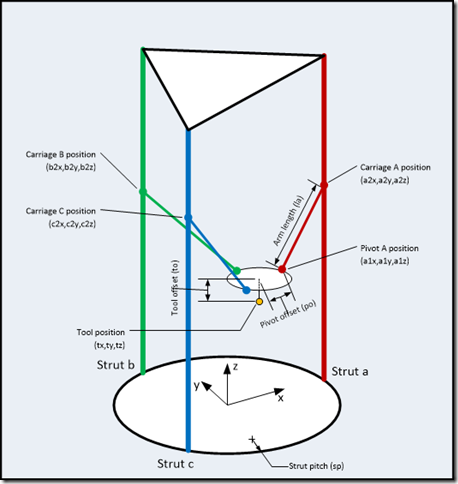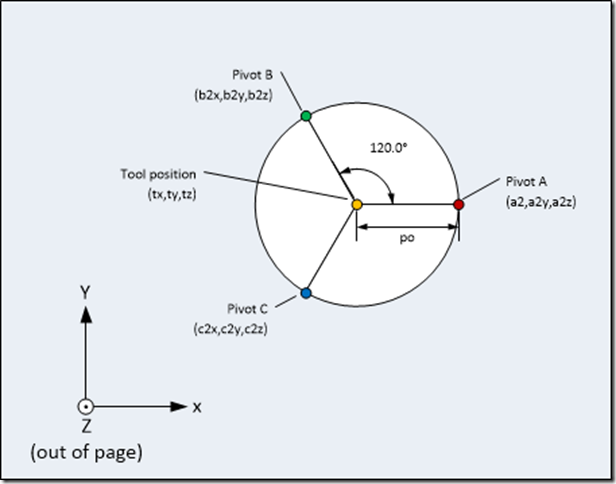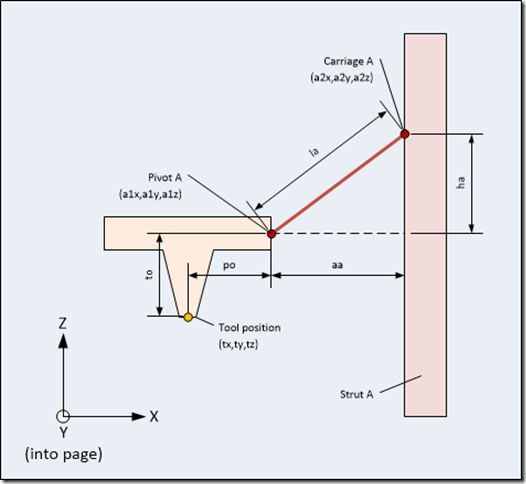# Scary maths

## Or how to convert from x,y,z coordinates to delta robot position

With a simple cartesian robot (or printer), the mechanism moves directly along rails in each of the x, y and z directions.  If you want to move a print head from the origin to, say, (10,10,20) you simply direct the motors to move it 10mm along the x axis rail, 10 along the y axis rail and 20 along the z.  You get the idea.  With a delta configuration, it’s not so simple.  Moving any one of the carriages which run up and down the three vertical struts will cause movement of the tool head in x,y and z simultaneously.  Calculations are required to work out how to move all three carriages at the same time to move the print head to the right place.  Fortunately, they are not too hard (and don’t need too much processor power for the Arduino to cope with).

Suppose we want to move the extruder nozzle to a particular point.  We’ll call this the tool position, and it will have coordinates (tx,ty,tz).  We want to calculate the positions of the three carriages (the bits that move up and down the struts) based on the tool head position.  We’ll call the carriages A, B and C.  Looking down from the top of the printer, Carriage A is on the X axis, carriage B is 120° anti-clockwise from A, and Carriage C is 240° anti-clockwise from A.  The positions of A, B and C are:

A2 = (a2x,a2y,a2z),
B2 = (b2x,b2y,b2z) and
C2 = (c2x,c2y,c2z).

The diagram below shows this a bit more clearly:Because we know that the arm length (la) is fixed and that the carriages can only move up and down (so we always know their x and y coordinates) we can calculate the carriage positions from some simple trigonometry.  First, we need to know the positions of the other ends of the arms (which I’m calling the the pivot points).  These are

A1 = (a1x,a1y,a1z),
B1 = (b1x,b1y,b1z) and
C1 = (c1x,c1y,c1z).

The diagram below shows how we can calculate these.  It’s a schematic view of the tool head, looking down from above.  We have to take into account the distance between the extruder nozzle and the pivot points, because it’s a finite size:Pivot A Pivot B Pivot C a1x = tx + poa1y = tya1z = tz + to b1x = tx + po * cos(120)b1y = ty + po * sin(120)b1z = tz + to c1x = tx + po * cos(240)c1y = ty + po * sin(240)c1z = tz + to

Once we know the locations of the pivot points, it’s straightforward to use Pythagoras’ theorem to calculate the distance in the x-y plane from the pivot to the carriage, and then use Pythagoras again to calculate the height the carriage must be above the pivot.  the next diagram shows the geometry, looking at it side-on:Firstly, we know the carriage head positions in x and y, because they are fixed to the strut positions:

 Carriage A Carriage B Carriage C a2x = spa2y = 0a2z = ? b2x = sp * cos(120)b1y = sp * sin(120)b2z = ? c2x = sp * cos(240)c2y = sp * sin(240)c2z=?

The x-y plane distances of the pivots to the struts are given by:

aa = sqrt((a2x-a1x)*(a2x-a1x) + (a2y-a1y)*(a2y-a1y))
ab = sqrt((b2x-b1x)*(b2x-b1x) + (b2y-b1y)*(b2y-b1y))
ac = sqrt((c2x-c1x)*(c2x-c1x) + (c2y-c1y)*(c2y-c1y))

And then the heights of the carriages above the pivot are calculated thus:

ha = sqrt((la*la) – (aa*aa))
hb = sqrt((la*la) – (ab*ab))
hc = sqrt((la*la) – (ac*ac))

so the heights of carriages above the floor of the printer (which is what we wanted to find), are then simply

a2z = tz + to + ha
b2z = tz + to + hb
c2z = tz + to + hc

And there it is.  The maths isn’t particularly hard, and I’ve described it in a procedural way to make it easy to implement in code should you so desire.  Calculating the other way (determining the nozzle position starting with the positions of the carriages) is harder, because it its necessary to calculate the intersection point of three spheres.  Fortunately, we don’t need to do that to drive a printer!

## 3 thoughts on “Scary maths”

1.Davey Dave Wurtzler says:

Absolutely spiffing description, but what about Newton-Raphson formulae?

Davey

1.peter says:

It’s certainly an efficient method of calculating the square root, especially if it’s being done repeatedly with slowly changing values (so you can use the previous value as a starting estimate for the next). I suspect that the marlin firmware I am using does it this way, but I don’t know for certain.

2.Angel says:

Excelente publicación. Gracias.